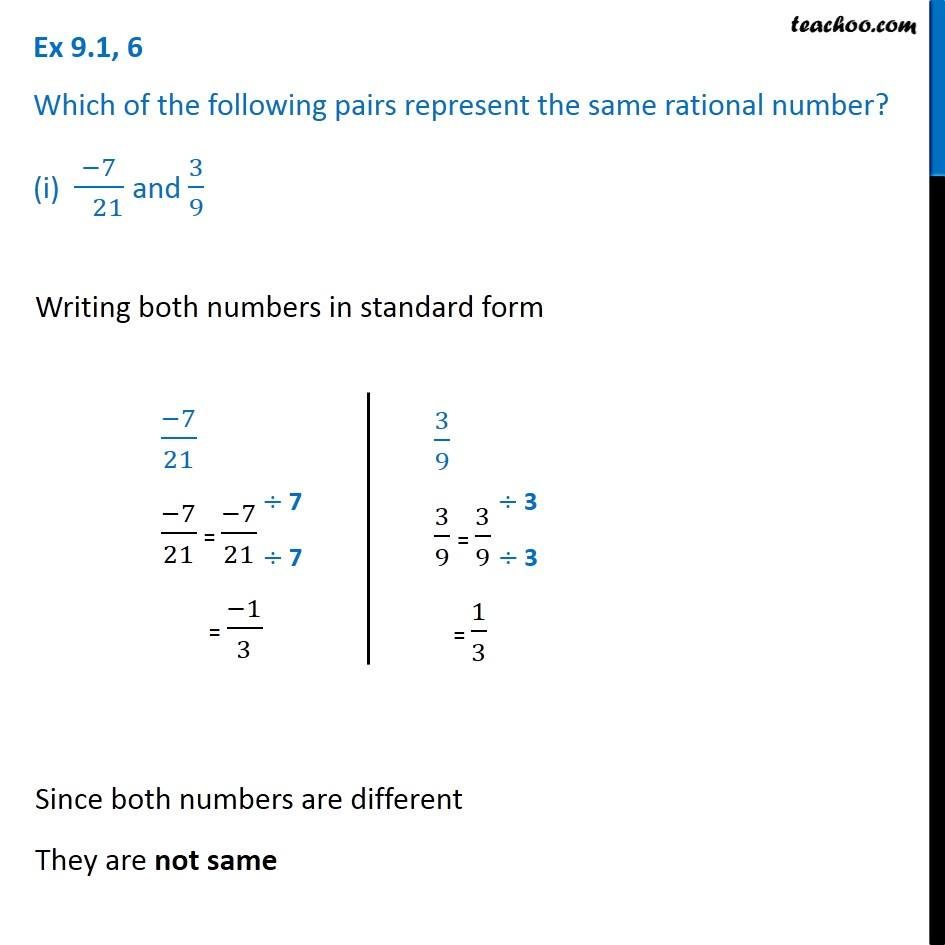Standard form of rational numbers

Chapter 8 Class 7 Rational Numbers
Concept wiseLearn in your speed, with individual attention - Teachoo Maths 1-on-1 Class

### Transcript

Ex 8.1, 6 Which of the following pairs represent the same rational number? (i) (−7)/( 21) and 3/9 Writing both numbers in standard form (−7)/21 (−7)/21 = (−7)/21 = (−1)/3 3/9 3/9 = 3/9 = 1/3 3/9 3/9 = 3/9 = 1/3 Since both numbers are different They are not same